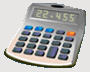DosageHelp.com Helping Nursing Students Prepare for Medication Exams By Explaining Dosage Calculations

# Fluid Maintenance Requirement Questions

Given the weight of a child or infant, calculate the necessary amount of fluid per day. Different hospitals may have different policies, but for learning how to perform these pediatric dosage calculations, the following commonly used table of fluid requirements may be used.

 Weight Range Required Daily Fluid 0-10 kg 100 mL per kg 10-20 kg 1,000 mL + 50 mL per each kg above 10 kg 20-70 kg 1,500 mL + 20 mL per each kg above 20 kg Over 70 kg 2,500 mL (adult requirement)

Example: An infant weighs 4 kg. What is the required amount of fluid per day in mL?
 0-10 kg 100 mL per kg
• 4 kg x 100 mL/kg = 400 mL

Example: An infant weighs 30.8 lb. What is the required IV flow rate in mL/hr to maintain proper fluid levels?
Convert 30.8 lb to kg.
• lb → kg    ( ÷ by 2.2 )
• 30.8 lb ÷ 2.2 = 14 kg
 10-20 kg 1,000 mL + 50 mL per each kg above 10kg
• 14 kg - 10 kg = 4 kg (There are 4 kg over 10 kg).
• 1,000 mL + (50 mL/kg x 4 kg) = 1,200 mL/day
 Volume (mL) Time (hr)
=  Y (Flow Rate in mL/hr)
There are 24 hours in one day.
• 1 day x 24 = 24 hr
 1,200 mL 24 hr
=  50 mL/hr

Home Dosage Question Steps Unit Conversion Mass for Mass Mass/Liquid For Liquid IV Terms & Abbreviations Amount in IV Fluid Volume/Time - IV mL Rate Volume/Time - IV Drop Rate Fluid Maintenance Dosage By Weight Mass/Time - IV mL Rate Practice Questions Question of the Day Tell a Friend Print a Flyer